# tkinter布局定位方法place讲解

### place方法的x,y参数

place()布局定位方法，允许程序员准确指定组件的位置，大小。粗略一听，好像不错，但实际操作起来，根本不容易，我们除了屏幕左上角x=0,y=0可以准确知道，其他位置并不能很好地知道坐标。至于组件大小，以像素为单位，同样不太好掌握，如果在界面上要操作的组件很好，组件之间的距离同样难很掌控。

这么一说，好像plaxe() 方法好像一无是处，那倒也不是，如果组件少，用place()方法也是可以的。我发现用这种方法用来把所有组件都定位在窗体的中间位置，是很方便，很容易的。

place(参数1,参数2,……)

```from tkinter import *
root=Tk()

but1=Button(root,text="五笔打字通")
but1.place(x=0,y=0)  # 第4行代码

root.mainloop()```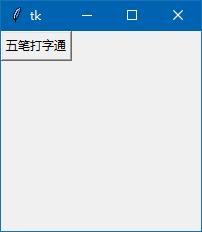```from tkinter import *
root=Tk()

img1=PhotoImage(file='zb.gif')   # 十字交叉图
la1=Label(root,image=img1,bd=0)
la1.place(x=0,y=0)

but1=Button(root,text="五笔打字通")
but1.place(x=100,y=100)  # 第4行代码

root.mainloop()```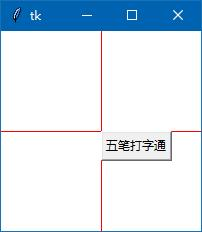### 锚点 anchor 讲解

anchor 翻译为“锚点”，这个参数决定组件以自身的那个一点去跟坐标相重合。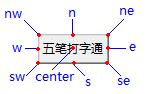```from tkinter import *
root=Tk()

img1=PhotoImage(file='zb.gif')
la1=Label(root,image=img1,bd=0)
la1.place(x=0,y=0)

but1=Button(root,text="五笔打字通")
but1.place(x=100,y=100,anchor='center')  # 第4行代码

root.mainloop()```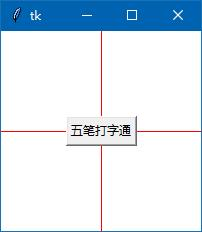### place方法的relx/rely参数

relx/rely 这也是一个坐标值 ，但是一个相对坐标，是相对父组件的坐标，取值范围为0-1，如：

…… 其实，特殊的位置都好计算，但其它位置就不太好计算了。

`but1.place(relx=0.5,rely=0.5,anchor='center')  # 第4行代码`

`but1.place(x=100,y=100,anchor='center')  # 第4行代码`

### place方法的width/height 和 relwidth/relheight 参数

width 指组件的宽度，单位是像素。

height 指组件的高度，单位是像素。

relwidth 指组件相对于父组件的宽度，取值范围为0-1

relheight指组件相对于父组件的高度，取值范围为0-1

### relx/rely 和x/y 可以组合在一起使用

`but1.place(relx=0.5,rely=0.5,x=25,y=25,anchor='center')  # 第4行代码`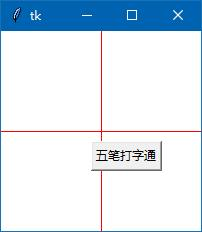### 让窗体的组件做为一个整体，居中显示

```from tkinter import *
root=Tk()
root.title('登录')

la1=Label(root,text='用户名：')

en1=Entry(root)  # 用户名文本框

la2=Label(root,text='密　码：')

en2=Entry(root)  # 密码文本框

but1=Button(root,text="确定")
but2=Button(root,text="取消")

root.resizable(False,False)
root.mainloop()```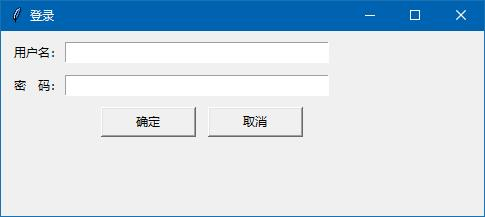1.  先去除倒数第2行  root.resizable(False,False) 代码

2.  在 root.title('登录') 下添加一个创建frame的代码，这个框架用relx/rely=0.5 锚点　anchor=’center’，让它始终处于窗体的正中心

```fr1=Frame(root,relief='ridge',borderwidth=1) # 不设置边线宽，无法显示
fr1.place(relx=0.5,rely=0.5,anchor='center')```

3.  然后，把下面的代码，6个组件的父组件从root 改成 fr1，这样，这6个组件都添加到fr1里，做为一个整体，窗体无论如何改变尺寸，它们始终处于窗体正中心。全部代码如下：

```from tkinter import *
root=Tk()
root.title('登录')

fr1=Frame(root,relief='ridge',borderwidth=1) # 不设置边线宽，无法显示
fr1.place(relx=0.5,rely=0.5,anchor='center')

la1=Label(fr1,text='用户名：')

en1=Entry(fr1)  # 用户名文本框

la2=Label(fr1,text='密　码：')

en2=Entry(fr1)  # 密码文本框

but1=Button(fr1,text="确定")
but2=Button(fr1,text="取消")

root.mainloop()```

```a,b=400,150
c=(root.winfo_screenwidth()-a)/2
d=(root.winfo_screenheight()-b)/2
root.geometry('%dx%d+%d+%d' % (a,b,c,d))```

`root.minsize(400,150)`

```from tkinter import *
root=Tk()
root.title('登录')

a,b=400,150
c=(root.winfo_screenwidth()-a)/2
d=(root.winfo_screenheight()-b)/2
root.geometry('%dx%d+%d+%d' % (a,b,c,d))

root.minsize(400,150)

fr1=Frame(root,relief='ridge',borderwidth=1) # 不设置边线宽，无法显示
fr1.place(relx=0.5,rely=0.5,anchor='center')

la1=Label(fr1,text='用户名：')

en1=Entry(fr1)  # 用户名文本框

la2=Label(fr1,text='密　码：')

en2=Entry(fr1)  # 密码文本框

but1=Button(fr1,text="确定")
but2=Button(fr1,text="取消")

root.mainloop()```

运行结果是，不管窗体如何调整尺寸，窗体上的组件始终处在窗体的正中间。

下篇文章，我们讲解一个我自己学习过程中的写的一个记事本的“关于”窗口代码，我认为只有运用自己学的知识，去写一些小的，实际有用的代码 ，才能巩固自己学习的知识。

<< 上一篇 下一篇 >>